Visualising Probability Distributions on the fx-CG50 - Casio Calculators

# Visualising Probability Distributions on the fx-CG50

Feb 2023 Medium Read: 5 MinAsk any teacher, and they’ll likely agree: displaying probability distributions has typically been a challenge on many graphing calculators. Fortunately, that’s all changing. With the new Distribution App on the fx-CG50, it’s now possible to visualise both discrete and continuous distributions with a graph. We spoke with experienced Maths Education Consultant Gerard Dummett to learn more about this big leap forward.

## How the Distribution App enhances probability visualisation

It’s well known that many graphic calculators have struggled to display probability distributions, particularly discrete probability distributions. But with the new Distribution App, the fx-CG50 overcomes this challenge and provides students with a clear visual representation, which they can explore and interrogate to improve their understanding.

The second big step forward are changes to how information is inputted. Gerard explains that an input screen was used to enter the parameters. Then, once inputted, students pressed the button and either saw a probability or, if in a continuous distribution, they also saw a graph. But if they wanted to change it, they had to go back to the input screen and start over again.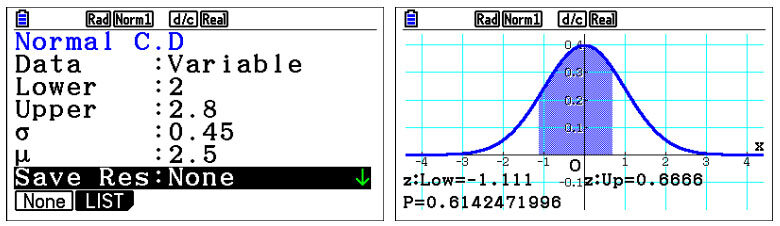Now, with the updated app, students are on the input screen; you’ve added the inputs and then want to change these inputs. You stay on the screen, and the input is immediately above where you are, immediately above the graph.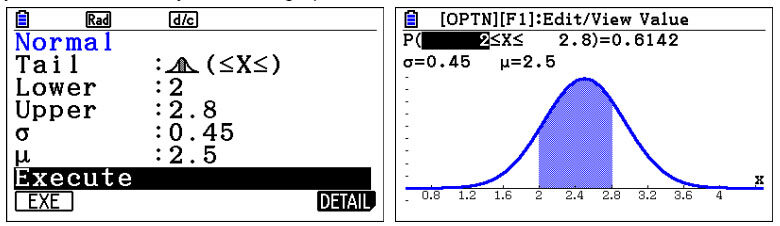## Exploring the teaching possibilities with the Distribution App

These two big improvements lead to many teaching possibilities. First, when dealing with a Normal distribution the visuals in the distribution app provide an ideal way for students to explore that symmetry.

Gerard explains with the example of standardised Normal distribution. In that case, if we go one standard deviation on either side of the mean, we can see an important property of the normal distribution, which is 68.3% of the area covered.They’ll also see it’s symmetrical. That’s quite important to an understanding of the normal distribution.

If you are dealing with the binomial distribution, by contrast, it is not generally symmetrical. It can be, but it typically is not. And so you want to see that values on either side of a given value might not be equal in the way they are in a normal distribution. And just getting the student to visualise that is quite important, and you can use it for teaching, which is one important advantage of the new distribution app.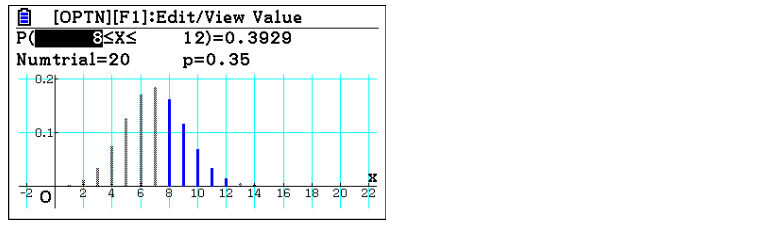### Ease of use gives students the confidence to explore

Another teaching point is the ease with which students can do things. For example, as Gerard explains, a question might ask the probability that the height of people in school falls within given bounds. If we’re looking at the distribution of that height, what is the probability between 1.48 and 1.53 metres, given that they are distributed in a particular way? Students can visualise the answer to that on the app.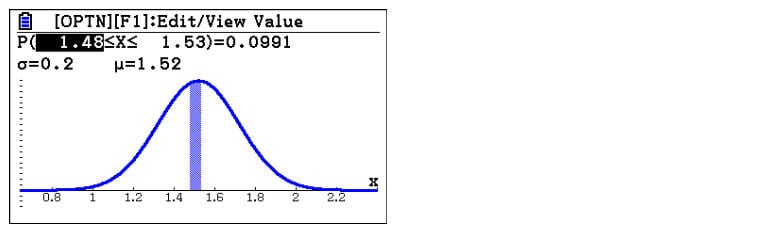Students could then explore what would happen if the value was 1.47 instead of 1.48. They can see what happens with that change, and the ease of use means students quickly build confidence when exploring new concepts.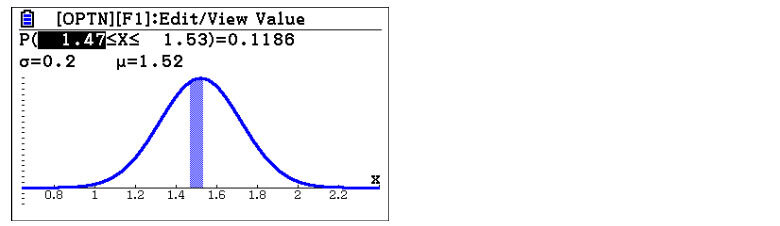They can then take it further, with more complicated questions. For instance, if the height is distributed equally on either side of the mean, what are the limits for us to cover 80% of students, which is 40% on either side of the mean? That is incredibly easy to do on the calculator now. Students type in your 80% in the probability; it returns the limits for you. The calculator draws the graph to show you how it looks, which is great for continuous distribution, especially for continuous symmetrical distributions.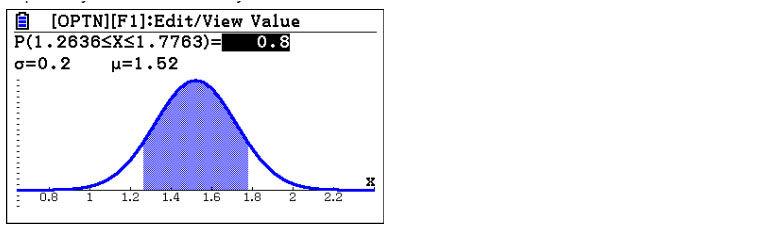But, with discrete distribution, where it might not be able to take the exact value you want to get that probability, the fact that it’s not continuous makes it harder because you’re not going to get the exact probability. The calculator helps here by finding the best probability between limits for students, and students can decide how to interpret that.

In this case, students will want to know the value of the variable that will cause the hypothesis to be either accepted or rejected. That value is typically 5% or 2.5%, but the problem is 5% or 2.5% may only correspond to a specific value if the distribution is continuous.

With discrete distributions, asking the calculator to provide the value of 5% means students can see in the visual representation if it’s too much or not. For example, if it comes out at 5.3% and they only wanted 5%, they’ll know they need to select a smaller value left tail) or larger value (right tail). In the case illustrated it has to be 12 instead of X being 11.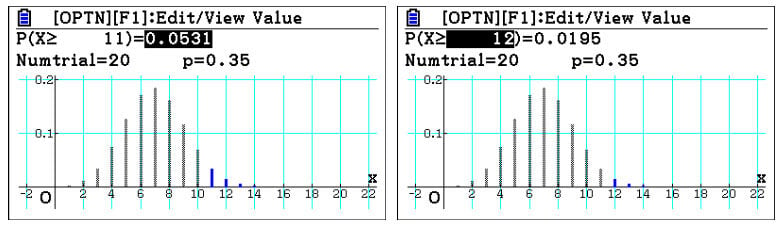On the diagram, this distinction is very obvious. It’s an area of maths that students often need help with; the ability to switch values, see the graph and interpret it is so powerful.

Are you looking to introduce the fx-CG50 in the classroom? We provide free skills training sessions to help you build confidence using graphic calculators in the classroom, which, in turn, will increase your students’ engagement. Sign up for your free session here!